# Current Electricity

##### Class 9th Science & Technology MHB Solution
Exercises
1. The accompanying figure shows some electrical appliances connected in a circuit in a…
2. The following figure shows the symbols for components used in the accompanying electrical…
3. Umesh has two bulbs having resistances of and He wants to connect them in a circuit, but…
4. The following table shows current in Amperes and potential difference in Volts. A. Find…
5. Match the pairs
6. The resistance of a conductor of length x is r. If its area of cross section is a, what is…
7. Resistances and are connected as shown in the figure. S1 and S2 are two keys. Discuss the…
8. Three resistances x1, x2 and x3 are connected in a circuit in different ways. X is the…
9. The resistance of a 1m long nichrome wire is 6 W. If we reduce the length of the wire to…
10. Solve the following problems. When two resistors are connected in series, their effective…
11. If a charge of 420 C flows through a conducting wire in 5 minutes what is the value of the…

###### Exercises
Question 1.

The accompanying figure shows some electrical appliances connected in a circuit in a house. Answer the following questions.

A. By which method are the appliances connected?

B. What must be the potential difference across individual appliances?

C. Will the current passing through each appliance be the same? Justify your answer.

D. Why are the domestic appliances connected in this way?

E. If the T.V. stops working, will the other appliances also stop working? Explain your answer.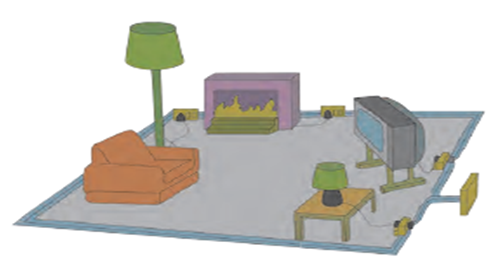A) The appliances are connected parallel to each other.

B) As the appliances are connected in parallel, the potential difference across individual appliances should be same. In India voltage ratings of all home appliances are constant i.e. 230V.

C) The current passing through each appliance won't be the same as the resistance of each appliance is different. (Current depends on both potential difference and resistance, I = V/R)

D) The domestic appliances are connected in this way as in a parallel circuit even if one of the appliance stops working, the rest would still be working but in case of series connection all the appliances stop working if one of them stops working.

E) If the TV stops working, the other appliances do not stop working as they are connected in parallel. In parallel connection, the potential difference between the loads is the same and the total current in the circuit is the sum of current flowing through each path in the circuit.

Question 2.

The following figure shows the symbols for components used in the accompanying electrical circuit. Place them at proper places and complete the circuit.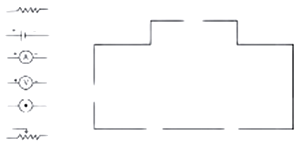Which law can you prove with the help of the above circuit?

The circuit with attached symbols is given below: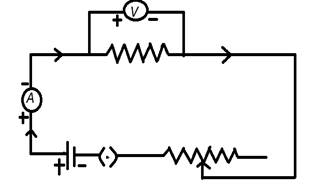Ohm’s law can be proved with the help of the above circuit.

Question 3.

Umesh has two bulbs having resistances of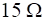and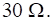He wants to connect them in a circuit, but if he connects them one at a time the filament gets burnt. Answer the following.

A. Which method should he use to connect the bulbs?

B. What are the characteristics of this way of connecting the bulbs depending on the answer of A above?

C. What will be the effective resistance in the above circuit?

Here we are assuming current to be constant and voltage to be varying.

A. The burning of filament is due to excessive heating when current passes through it. More the resistance of the wire, more is the heating. Therefore, the resistance of the circuit should be decreased which can be done if both the bulbs are connected in parallel.

We know that equivalent resistance in case of parallel combination of two resistances R1 and R2 is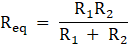In the above case,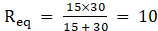Ω which is less than the individual resistances of both the wires.

Therefore, both the bulbs should be connected in parallel.

B. Characteristics of a parallel circuit:

1) A parallel circuit has two or more paths for current to flow through and the total current is equal to sum of currents flowing through these paths.

2) Voltage is same across each component of parallel circuit.

3) The current in all the branches is same. As shown in the figure: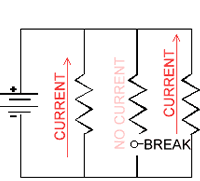C. The effective resistance in the above circuit is given as: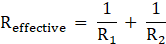Where R1 and R2 are the resistances connected in parallel.

or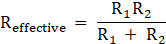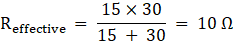Question 4.

The following table shows current in Amperes and potential difference in Volts.

A. Find the average resistance.

B. What will be the nature of the graph between the current and potential difference? (Do not draw a graph.)

C. Which law will the graph prove?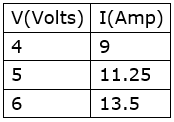A. According to the equation V = IR

R = V/I

Resistance in the 1st case R1 = V1/I1

= 4/9

= 0.444 Ω

Resistance in the 2nd case R2 = V2/I2

= 5/11.25

= 0.444 Ω

Resistance in the 3rd case R3 = V3/I3

= 6/13.5

= 0.444 Ω

Average resistance Ravg =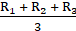= (0.444 + 0.444 + 0444)/3

= 0.444 Ω

B. In this case as R is constant, we can observe the increase in I as V increases.

We know that V = IR .

It represents a straight line starting from origin with a slope R. Therefore, the nature of graph between current and potential difference will be a straight line.

The graph is given below: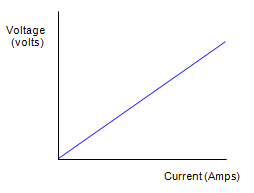C. The graph proves Ohm's law.

According to Ohm's law the current passing through a conductor between two points is directly proportional to the voltage across the two points.

I∝V

I = V/R

Question 5.

Match the pairs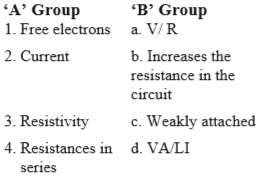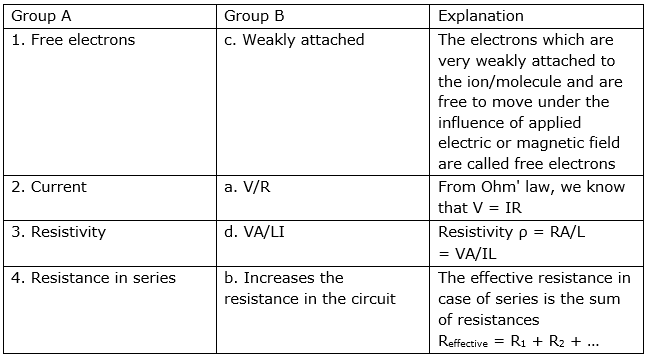Question 6.

The resistance of a conductor of length x is r. If its area of cross section is a, what is its resistivity? What is its unit?

We know that Resistivity ρ = RA/L

where R = Resistance of the conductor

A = Cross sectional area of the conductor

L = Length of the conductor

Here A = a and L = x

Therefore, Resistivity ρ = Ra/x

It's units are ohm.m2/m = Ωm

Question 7.

Resistances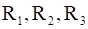and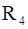are connected as shown in the figure. S1 and S2 are two keys. Discuss the current flowing in the circuit in the following cases.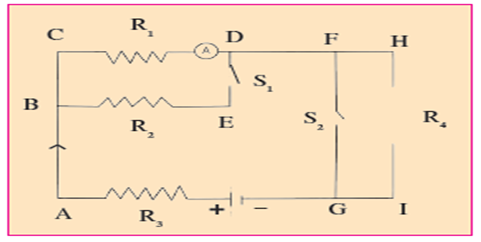A. Both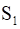and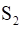are closed.

B. Bothandare open.

C.is closed butis open

A. When both S1 and S2 are closed, R4 is short circuited.

R3 and (R1||R2) are in series

Effective resistance of the circuit Reffective =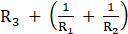⇒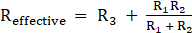Let the applied voltage be V.

Current in the circuit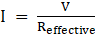⇒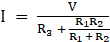B. When both S1 and S2 are open, R3 , R1 and R4 are in series.

The effective resistance of the circuit,

Reffective = R3 + R1 + R4

Let the applied voltage be V.

Current in the circuit I = V/Reffective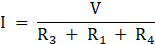C. When S1 is closedbut S2 is open, R3, (R1||R2) and R4 are in series.

As S1 is closed, R1 and R2 are parallel to each other.

The effective resistance of the circuit is

Reffective = R3 + (R1||R2) + R4 ( When R1||R2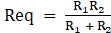)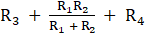Let the applied voltage be V

Current in the circuit I = V/Reffective

I =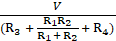Question 8.

Three resistances x1, x2 and x3 are connected in a circuit in different ways. X is the effective resistance. The properties observed for these different ways of connecting x1, x2 and x3 are given below. Write the way in which they are connected in each case. (I-current, V-potential difference, x-effective resistance)

A. Current I-flows through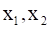and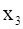B. x is larger thanandC. x is smaller thanandD. The potential acrossandis the same

E.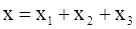F.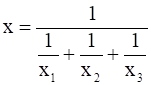A. The same current flows through all the three conductors x1, x2 and x3. So, all the three must be connected in series.

Because in series connection, the current in all the wires is same.

B. Effective resistance X is larger than x1, x2 and x3 individually.

The only way in which this can be possible is if all the three conductors are connected in series.

In series combination of resistances,

Reffective = R1 + R2 + R3

Therefore, the effective resistance is greater than the resistances taken individually.

C. Effective resistance X is smaller than x1 , x2 and x3 taken individually. This can happen only when the three resistances are connected in parallel.

In parallel combination of resistances,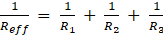Therefore, the effective resistance reduces when resistances are connected parallel to each other.

D. The potential across the conductors is same when they are connected in parallel.

E.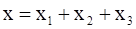is the formula for calculating effective resistance of a circuit when conductors are connected in series

F.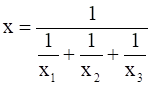is the formula for calculating effective resistance of a circuit when conductors are connected in parallel.

Question 9.

Solve the following problems.

The resistance of a 1m long nichrome wire is 6 W. If we reduce the length of the wire to 70 cm. what will its resistance be?

Given:

Resistance of a 1m(100cm) long(L1) nichrome wire = 6 Ω (R1)

We know that resistance is directly proportional to length of the

conductor.

Therefore R1/R2 = L1/L2

6/R2 = 100/70

R2 = 4.2 Ω

Question 10.

Solve the following problems.
When two resistors are connected in series, their effective resistance is 80 Ω. When they are connected in parallel, their effective resistance is 20 Ω. What are the values of the two resistances?

Given:

Effective resistance when two resistors are connected in series =80 Ω

Let us consider the resistances as R1 and Rin series combination,

V = V+ Vand V=IR

As we know that in series connection current is constant, we get

R= R1 + R2 = 80 Ω ---(1)

Effective resistance when two resistors are connected in parallel combination = 20Ω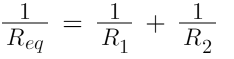We get........(2)

putting the value of equation(1) in equation(2)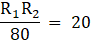R1R2 = 1600

(R1-R2)2 = (R1 + R2)2 - 4R1R2

= 802 - (4×1600)

= 0

R1-R2 = 0 ---(3)

R1=40

Subtracting (1) and(3)

R2=40Ω

we get R1 = R2 = 40Ω

Question 11.

Solve the following problems.

If a charge of 420 C flows through a conducting wire in 5 minutes what is the value of the current?

Given:

Charge (q) = 420C

Time for which the charge flows (t) = 5 min = 300 s

We know that current I = q/t

= 420/300

= 1.4 A

Therefore current flowing through the conductor is 1.4 A.

## PDF FILE TO YOUR EMAIL IMMEDIATELY PURCHASE NOTES & PAPER SOLUTION. @ Rs. 50/- each (GST extra)

SUBJECTS

HINDI ENTIRE PAPER SOLUTION

MARATHI PAPER SOLUTION
SSC MATHS I PAPER SOLUTION
SSC MATHS II PAPER SOLUTION
SSC SCIENCE I PAPER SOLUTION
SSC SCIENCE II PAPER SOLUTION
SSC ENGLISH PAPER SOLUTION
SSC & HSC ENGLISH WRITING SKILL
HSC ACCOUNTS NOTES
HSC OCM NOTES
HSC ECONOMICS NOTES
HSC SECRETARIAL PRACTICE NOTES

2019 Board Paper Solution

HSC ENGLISH SET A 2019 21st February, 2019

HSC ENGLISH SET B 2019 21st February, 2019

HSC ENGLISH SET C 2019 21st February, 2019

HSC ENGLISH SET D 2019 21st February, 2019

SECRETARIAL PRACTICE (S.P) 2019 25th February, 2019

HSC XII PHYSICS 2019 25th February, 2019

CHEMISTRY XII HSC SOLUTION 27th, February, 2019

OCM PAPER SOLUTION 2019 27th, February, 2019

HSC MATHS PAPER SOLUTION COMMERCE, 2nd March, 2019

HSC MATHS PAPER SOLUTION SCIENCE 2nd, March, 2019

SSC ENGLISH STD 10 5TH MARCH, 2019.

HSC XII ACCOUNTS 2019 6th March, 2019

HSC XII BIOLOGY 2019 6TH March, 2019

HSC XII ECONOMICS 9Th March 2019

SSC Maths I March 2019 Solution 10th Standard11th, March, 2019

SSC MATHS II MARCH 2019 SOLUTION 10TH STD.13th March, 2019

SSC SCIENCE I MARCH 2019 SOLUTION 10TH STD. 15th March, 2019.

SSC SCIENCE II MARCH 2019 SOLUTION 10TH STD. 18th March, 2019.

SSC SOCIAL SCIENCE I MARCH 2019 SOLUTION20th March, 2019

SSC SOCIAL SCIENCE II MARCH 2019 SOLUTION, 22nd March, 2019

XII CBSE - BOARD - MARCH - 2019 ENGLISH - QP + SOLUTIONS, 2nd March, 2019

HSC Maharashtra Board Papers 2020

(Std 12th English Medium)

HSC ECONOMICS MARCH 2020

HSC OCM MARCH 2020

HSC ACCOUNTS MARCH 2020

HSC S.P. MARCH 2020

HSC ENGLISH MARCH 2020

HSC HINDI MARCH 2020

HSC MARATHI MARCH 2020

HSC MATHS MARCH 2020

SSC Maharashtra Board Papers 2020

(Std 10th English Medium)

English MARCH 2020

HindI MARCH 2020

Hindi (Composite) MARCH 2020

Marathi MARCH 2020

Mathematics (Paper 1) MARCH 2020

Mathematics (Paper 2) MARCH 2020

Sanskrit MARCH 2020

Important-formula

THANKS